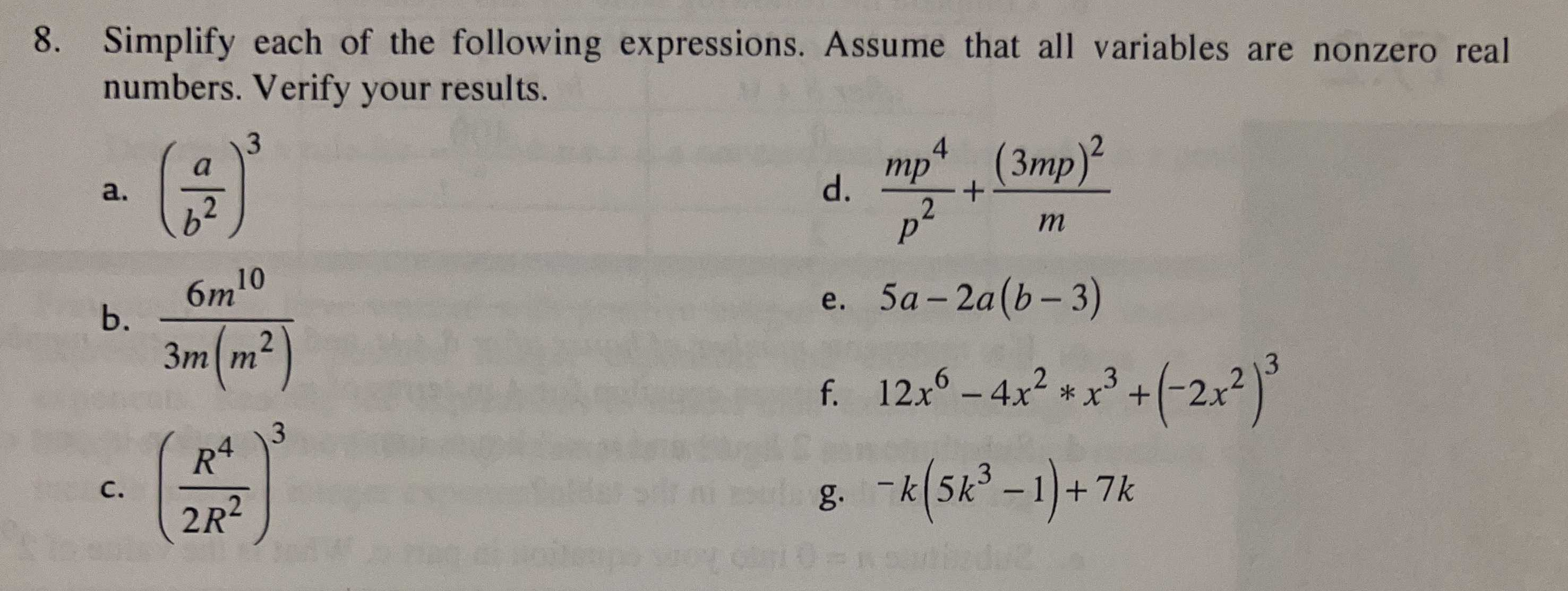### ¿Todavía tienes preguntas de matemáticas?

Pregunte a nuestros tutores expertos
Algebra
Pregunta8. Simplify each of the following expressions. Assume that all variables are nonzero real numbers. Verify your results. a. $$( \frac { a } { b ^ { 2 } } ) ^ { 3 }$$ d. $$\frac { m p ^ { 4 } } { p ^ { 2 } } + \frac { ( 3 m p ) ^ { 2 } } { m }$$

b. $$\frac { 6 m ^ { 10 } } { 3 m ( m ^ { 2 } ) }$$ e. $$5 a - 2 a ( b - 3 )$$

c. $$( \frac { R ^ { 4 } } { 2 R ^ { 2 } } ) ^ { 3 }$$ f. $$12 x ^ { 6 } - 4 x ^ { 2 } * x ^ { 3 } + ( - 2 x ^ { 2 } ) ^ { 3 }$$

g. $$- k ( 5 k ^ { 3 } - 1 ) + 7 k$$ d